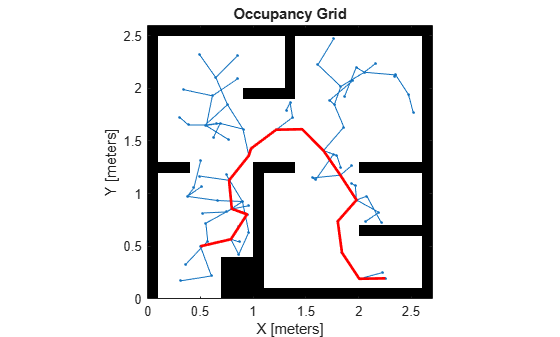stateSpaceSE2

SE(2) state space

Description

The stateSpaceSE2 object stores parameters and states in the SE(2) state space, which is composed of state vectors represented by [x, y, θ]. x and y are Cartesian coordinates, and θ is the orientation angle. The object uses Euclidean distance to calculate distance and uses linear interpolation to calculate translation and rotation of the state.

Creation

Description

example

space = stateSpaceSE2 creates an SE(2) state space object with default state bounds for x, y, and θ.

space = stateSpaceSE2(bounds) specifies the bounds for x, y, and θ. The state values beyond the bounds are truncated to the bounds. The input, bounds, allows you to set the value of the StateBounds property.

Properties

expand all

Name of state space, specified as a string.

Dimension of the state space, specified as a positive integer.

Bounds of state variables, specified as a 3-by-2 real-valued matrix.

• The first row specifies the lower and upper bounds of the x state in meters.

• The second row specifies the lower and upper bounds of the y state in meters.

• The third row specifies the lower and upper bounds of the θ state in radians.

Data Types: double

Weight applied to x and y distance calculation, specified as a nonnegative real scalar.

In the object, the distance calculated as:

$d=\sqrt{\left({w}_{xy}\left({d}_{x}^{2}+{d}_{y}^{2}\right)\right)+{w}_{\theta }{d}_{\theta }^{2}}$

wxy is weight applied to x and y coordinates, and wθ is the weight applied to the θ coordinate. dx, dy, and dθ are the distances in the x, y, and θ direction, respectively.

Data Types: double

Weight applied to θ distance calculation, specified as a nonnegative real scalar.

In the object, the distance calculated as:

$d=\sqrt{\left({w}_{xy}\left({d}_{x}^{2}+{d}_{y}^{2}\right)\right)+{w}_{\theta }{d}_{\theta }^{2}}$

wxy is weight applied to x and y coordinates, and wθ is the weight applied to the θ coordinate. dx, dy, and dθ are the distances in the x, y, and θ direction, respectively.

Data Types: double

Object Functions

 copy Create deep copy of state space object distance Distance between two states enforceStateBounds Reduce state to state bounds interpolate Interpolate between states sampleGaussian Sample state using Gaussian distribution sampleUniform Sample state using uniform distribution

Examples

collapse all

Create an SE(2) state space.

ss = stateSpaceSE2;

Create an occupancyMap-based state validator using the created state space.

sv = validatorOccupancyMap(ss);

Create an occupancy map from an example map and set map resolution as 10 cells/meter.

map = occupancyMap(simpleMap,10);
sv.Map = map;

Set validation distance for the validator.

sv.ValidationDistance = 0.01;

Update state space bounds to be the same as map limits.

ss.StateBounds = [map.XWorldLimits;map.YWorldLimits; [-pi pi]];

Create the path planner and increase maximum connection distance.

planner = plannerRRT(ss,sv);
planner.MaxConnectionDistance = 0.3;

Set the start and goal states.

start = [0.5,0.5,0];
goal = [2.5,0.2,0];

Plan a path with default settings.

rng(100,'twister'); % for repeatable result
[pthObj,solnInfo] = planner.plan(start,goal);

Visualize the results.

map.show; hold on;
plot(solnInfo.TreeData(:,1),solnInfo.TreeData(:,2),'.-'); % tree expansion
plot(pthObj.States(:,1), pthObj.States(:,2),'r-','LineWidth',2) % draw path